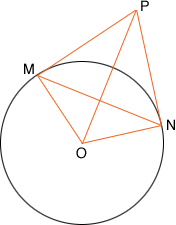Quandaries and Queries Hello. My name is Tej, i am a student of the tenth standard from India. This is a secondary level problem I cannot manage to solve. Please help! The problem is----- The tangents drawn from points M and N of a circle having centre O intersect each other at point P. If angle MPN=60 degrees, NM=10, then find the radius of the circle and Area of quadrilateral OMPN. Hi Tej, I drew the diagram you described.If you can show that triangles PON and PMO are congruent then angles PMN and MNP are equal and thus must each measure 60 degrees. Thus triangle PMN is an equilateral triangle. Does this help? Penny Go to Math Central